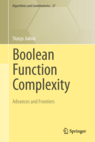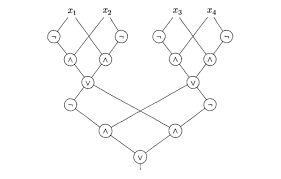Go to the roots of calculations! Group the operations. Classify them according to their complexities rather than their appearances! This, I believe, is the mission of future mathematicians. - Evariste Galois

## What is circuit complexity about?

In theoretical computer science, circuit complexity is a branch of computational complexity theory in which Boolean functions are classified according to the size or depth of the Boolean circuits that compute them. The main mission of circuit complexity is to understand why some Boolean functions require much larger circuits than other Boolean functions. Lower-bounding the size of circuits is also a purely "pragmatic" problem in the design of computer chips and in cryptography. Proving circuit lower bounds is also a possible approach to separate complexity classes such as P and NP.

Boolean (or switching) functions map each sequence of bits to a single bit $0$ or $1$. Bit $0$ is usually interpreted as false'', and bit $1$ as true''. The simplest of such functions are the And $x\cdot y$, non-exclusive Or $x\lor y$, negation $\neg x=1-x$.Fig.1 A Boolean circuit over the basis $\{\lor,\land,\neg\}$ with 15 gates. The circuit outputs $1$ iff either $x_1=x_2$ and $x_3\neq x_4$ or $x_1\neq x_2$ and $x_3=x_4$, that is, if the sum $x_1+x_2+x_3+x_4$ is either $1$ or $3$. The central problem of Boolean function complexity---the lower bounds problem---is:
Lower Bounds Problem: Given a boolean function, how many of these simplest operations do we need to compute the function on all input vectors?
The difficulty in proving that a given boolean function has high complexity lies in the nature of our adversary: the circuit. Small circuits may work in a counterintuitive fashion, using deep, devious, and fiendishly clever ideas. How can one prove that there is no clever way to quickly compute the function? This is the main issue confronting complexity theorists. The problem lies on the border between mathematics and computer science: lower bounds are of great importance for computer science, but their proofs require techniques from combinatorics, algebra, analysis, probability theory, and other branches of mathematics.

My book "Boolean Function Complexity" is all about the lower bounds problem.